#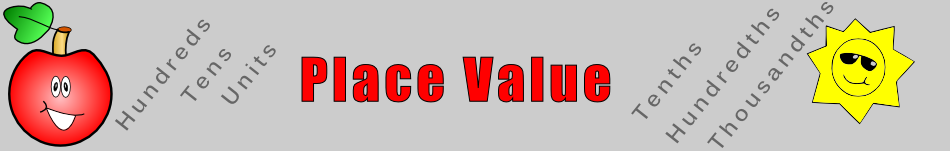There are 366 different Starters of The Day, many to choose from. You will find below some starters on the topic of Place Value. A lesson starter does not have to be on the same topic as the main part of the lesson or the topic of the previous lesson. It is often very useful to revise or explore other concepts by using a starter based on a totally different area of Mathematics.

Main Page

### Place Value Starters:Develop a quick way of mentally multiplying any number by 1001.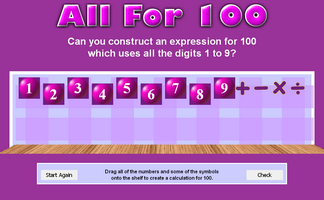Can you write an expression for 100 which uses all the digits 1 to 9?Use only the 1, 5 and 0 keys on a calculator to make given totals.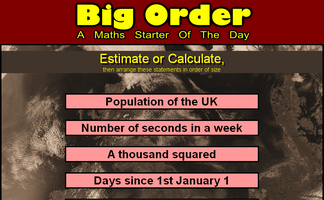Estimate or calculate then put the large numbers in order of size.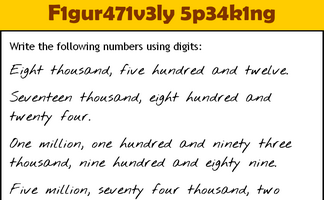Write the numbers written as words using digits.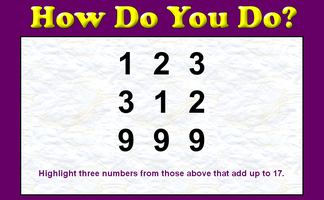Without a calculator perform some calculations requiring a knowledge of place value.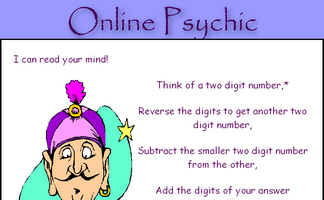Work out why subtracting a two digit number from its reverse gives a multiple of nine.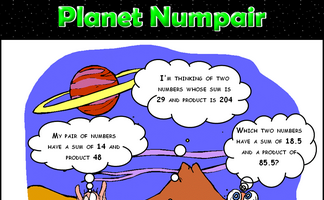Arrange the digits one to nine to make a correct addition calculation.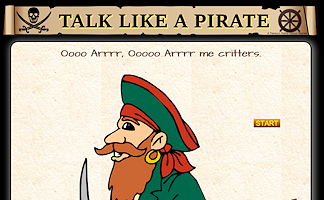Write out in words some numbers writen as digits (optional pirate theme)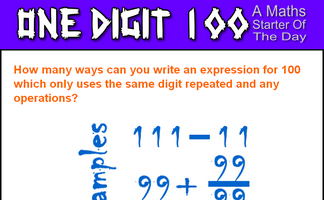How many ways can you write an expression for 100 which only uses the same digit repeated and any operations?Arrange the numbers to produce the largest product.Find a calculation for the current year which uses all of the digits 1 to 9.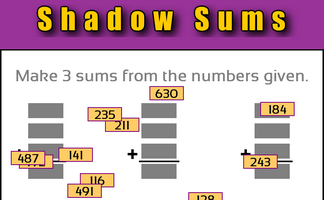Make sums from the three digit numbers given.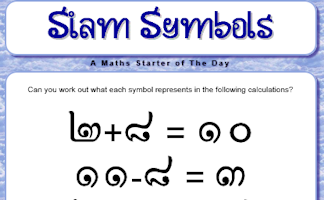Can you work out what each of the strange symbols represents in these calculations?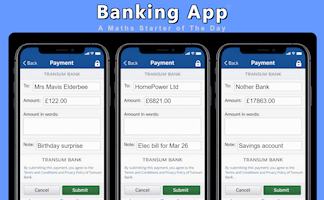Complete some imaginary cheques, the amount needs to be written in words.Find calculations which written back to front give the same answer.

## Exercises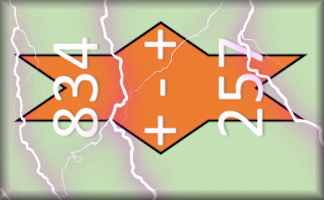#### Awe-Sum

Arrange the given digits to make six 3-digit numbers that combine in an awesome way.#### Centexpression

Arrange the numbers from 1 to 9 to make an expression with a value of 100.#### Countdown

How close can you get to the target by making a calculation out of the five numbers given?#### Decimals Line

A number line showing tenths and hundredths with draggable arrows. This is a visual aid designed to be projected onto a whiteboard for whole class exposition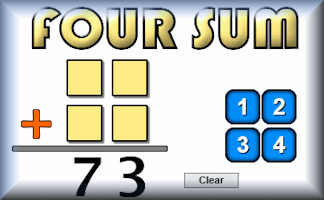#### Four Sum

Arrange the given number tiles to make two 2 digit numbers that add up to the given total.#### Great Expectation

An interactive online activity requiring logical thinking and a certain amount of luck to place the digits on the correct side of the inequality sign.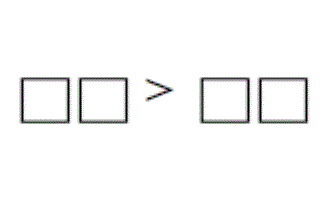#### Greater Than

The teacher has a set of six cards numbered 1 to 6. They are placed face down on the teachers desk so that the teacher can pick up one at random which students then have to fit onto a grid.Without a calculator perform some calculations requiring a knowledge of place value.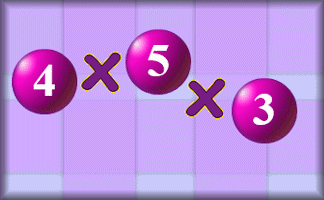#### Largest Product

A drag and drop activity challenging you to arrange the digits to produce the largest possible product.#### Numbers in Words

Find the five lettered mathematical words by matching numbers with their equivalent in words.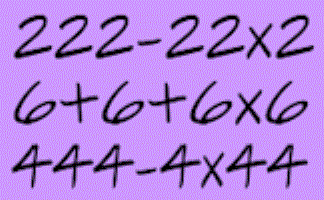#### One Digit Only

Find expressions using only one digit which equal the given targets.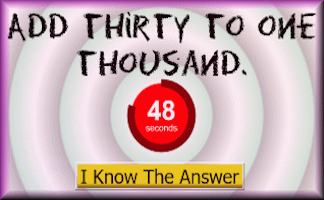#### One Minute Maths

A challenge to mentally add numbers together without making the classic place value mistakes.#### Online Psychic

Let the psychic read the cards and magically reveal the number you have secretly chosen. What is the mathematics that makes this trick work?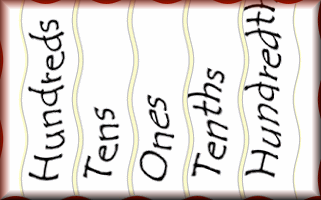#### Place Value

Do this online exercise to show you understand place value for decimals and integers of any size.#### Place Value Chart

This is a visual aid designed to be projected onto a whiteboard for whole class exposition about place value.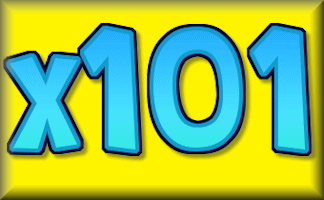#### Quick

Can you multiply a number by 1001 in your head? This exercise provides practice in this and other similar challenges.#### Rounding SF

A self marking exercise requiring students to round numbers to a given number of significant figures.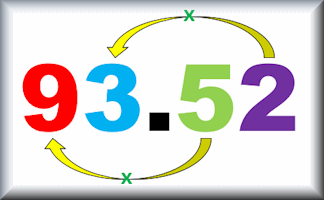#### The Value of Places

Test your understanding of place value by comparing the values of digits in different positions within numbers.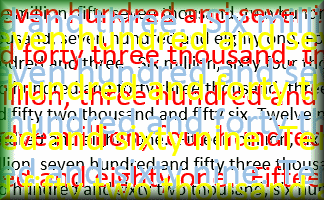#### Words in Digits

Write the numbers given in words as digits and vice versa.

### Search

The activity you are looking for may have been classified in a different way from the way you were expecting. You can search the whole of Transum Maths by using the box below.

Have today's Starter of the Day as your default homepage. Copy the URL below then select
Tools > Internet Options (Internet Explorer) then paste the URL into the homepage field.

Set as your homepage (if you are using Internet Explorer)

Do you have any comments? It is always useful to receive feedback and helps make this free resource even more useful for those learning Mathematics anywhere in the world. Click here to enter your comments.For All: